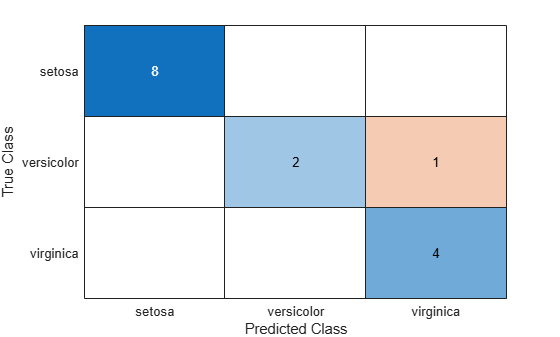# predict

Classify observations using ensemble of classification models

## Syntax

``labels = predict(Mdl,X)``
``labels = predict(Mdl,X,Name,Value)``
``````[labels,score] = predict(___)``````

## Description

````labels = predict(Mdl,X)` returns a vector of predicted class labels for the predictor data in the table or matrix `X`, based on the full or compact, trained classification ensemble `Mdl`.```
````labels = predict(Mdl,X,Name,Value)` uses additional options specified by one or more `Name,Value` pair arguments.```
``````[labels,score] = predict(___)``` also returns a matrix of classification scores (`score`), indicating the likelihood that a label comes from a particular class, using any of the input arguments in the previous syntaxes. For each observation in `X`, the predicted class label corresponds to the maximum score among all classes.```

## Input Arguments

 `Mdl` A classification ensemble created by `fitcensemble` or a compact classification ensemble created by `compact`. `X` Predictor data to be classified, specified as a numeric matrix or table. Each row of `X` corresponds to one observation, and each column corresponds to one variable. For a numeric matrix: The variables making up the columns of `X` must have the same order as the predictor variables that trained `Mdl`.If you trained `Mdl` using a table (for example, `Tbl`), then `X` can be a numeric matrix if `Tbl` contains all numeric predictor variables. To treat numeric predictors in `Tbl` as categorical during training, identify categorical predictors using the `CategoricalPredictors` name-value pair argument of `fitcensemble`. If `Tbl` contains heterogeneous predictor variables (for example, numeric and categorical data types) and `X` is a numeric matrix, then `predict` throws an error. For a table: `predict` does not support multi-column variables and cell arrays other than cell arrays of character vectors.If you trained `Mdl` using a table (for example, `Tbl`), then all predictor variables in `X` must have the same variable names and be of the same data types as those that trained `Mdl` (stored in `Mdl.PredictorNames`). However, the column order of `X` does not need to correspond to the column order of `Tbl`. `Tbl` and `X` can contain additional variables (response variables, observation weights, etc.), but `predict` ignores them.If you trained `Mdl` using a numeric matrix, then the predictor names in `Mdl.PredictorNames` and corresponding predictor variable names in `X` must be the same. To specify predictor names during training, see the `PredictorNames` name-value pair argument of `fitcensemble`. All predictor variables in `X` must be numeric vectors. `X` can contain additional variables (response variables, observation weights, etc.), but `predict` ignores them.

### Name-Value Pair Arguments

Specify optional comma-separated pairs of `Name,Value` arguments. `Name` is the argument name and `Value` is the corresponding value. `Name` must appear inside quotes. You can specify several name and value pair arguments in any order as `Name1,Value1,...,NameN,ValueN`.

 `'Learners'` Indices of weak learners `predict` uses for computation of responses, a numeric vector. Default: `1:T`, where `T` is the number of weak learners in `Mdl` `'UseObsForLearner'` A logical matrix of size `N`-by-`T`, where: `N` is the number of rows of `X`.`T` is the number of weak learners in `Mdl`. When `UseObsForLearner(i,j)` is `true`, learner `j` is used in predicting the class of row `i` of `X`. Default: `true(N,T)`

## Output Arguments

 `labels` Vector of classification labels. `labels` has the same data type as the labels used in training `Mdl`. (The software treats string arrays as cell arrays of character vectors.) `score` A matrix with one row per observation and one column per class. For each observation and each class, the score generated by each tree is the probability of this observation originating from this class computed as the fraction of observations of this class in a tree leaf. `predict` averages these scores over all trees in the ensemble.

## Examples

expand all

Load Fisher's iris data set. Determine the sample size.

```load fisheriris N = size(meas,1);```

Partition the data into training and test sets. Hold out 10% of the data for testing.

```rng(1); % For reproducibility cvp = cvpartition(N,'Holdout',0.1); idxTrn = training(cvp); % Training set indices idxTest = test(cvp); % Test set indices```

Store the training data in a table.

```tblTrn = array2table(meas(idxTrn,:)); tblTrn.Y = species(idxTrn);```

Train a classification ensemble using AdaBoostM2 and the training set. Specify tree stumps as the weak learners.

```t = templateTree('MaxNumSplits',1); Mdl = fitcensemble(tblTrn,'Y','Method','AdaBoostM2','Learners',t);```

Predict labels for the test set. You trained model using a table of data, but you can predict labels using a matrix.

`labels = predict(Mdl,meas(idxTest,:));`

Construct a confusion matrix for the test set.

`confusionchart(species(idxTest),labels)``Mdl` misclassifies one versicolor iris as virginica in the test set.

expand all